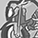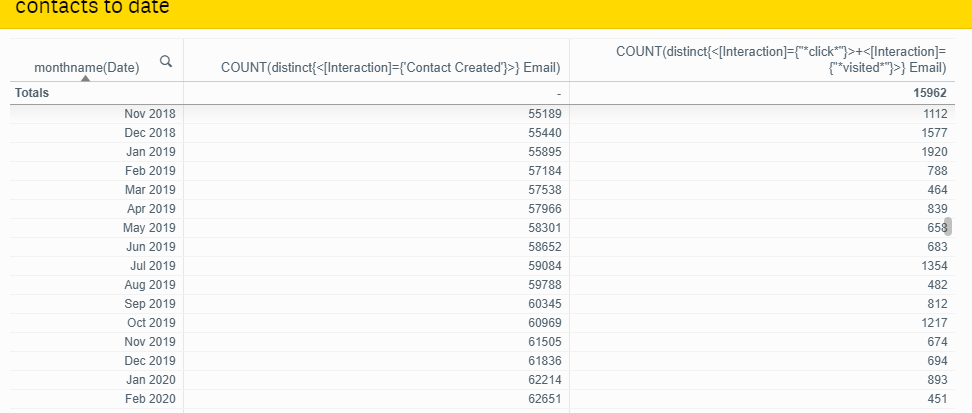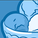# App Development

Announcements
April 22, 2PM EST: Learn about GeoOperations in Qlik Sense SaaS READ MORE
cancel
Showing results for
Did you mean:Contributor

## Cumulative Sum Across Date Output Expression Not Working

Hi  - I've got a chart where I've used the modifier to show the cumulative total by month of the set analysis. I need to do some further calculations on that number by month but the output expression doesn't work. Does anyone know how I can replicate what the chart/modifier is doing?The end result I'm looking for is to be able to divide the 2nd column by the 3rd

Thanks

Labels (4)

• ### Set Analysis

2 Solutions

Accepted SolutionsSpecialist

You can use the rangesum function to get similar results as using Modifier->Accumulation.  Something like:

rangesum(above(total Count(distinct {<[Interaction]={'Contact Created'}>} Email), 0, rowno(Total))) /

Count(distinct {<[Interaction]={'click'}>+<[Interaction]={'visited'}>} Email)Specialist

Sorry, I missed the closing '}' on the set analysis in the first expression.  Try this:

rangesum(above(total Count(distinct {<[Interaction]={'Contact Created'}>} Email), 0, rowno(Total))) /

Count(distinct {<[Interaction]={'click'}>+<[Interaction]={'visited'}>} Email)

4 RepliesSpecialist

You can use the rangesum function to get similar results as using Modifier->Accumulation.  Something like:

rangesum(above(total Count(distinct {<[Interaction]={'Contact Created'}>} Email), 0, rowno(Total))) /

Count(distinct {<[Interaction]={'click'}>+<[Interaction]={'visited'}>} Email)Contributor
Author

hmmm getting a missing ( errorSpecialist

Sorry, I missed the closing '}' on the set analysis in the first expression.  Try this:

rangesum(above(total Count(distinct {<[Interaction]={'Contact Created'}>} Email), 0, rowno(Total))) /

Count(distinct {<[Interaction]={'click'}>+<[Interaction]={'visited'}>} Email)Contributor
Author

Not sure why I was - working good!Tags
Community Browser